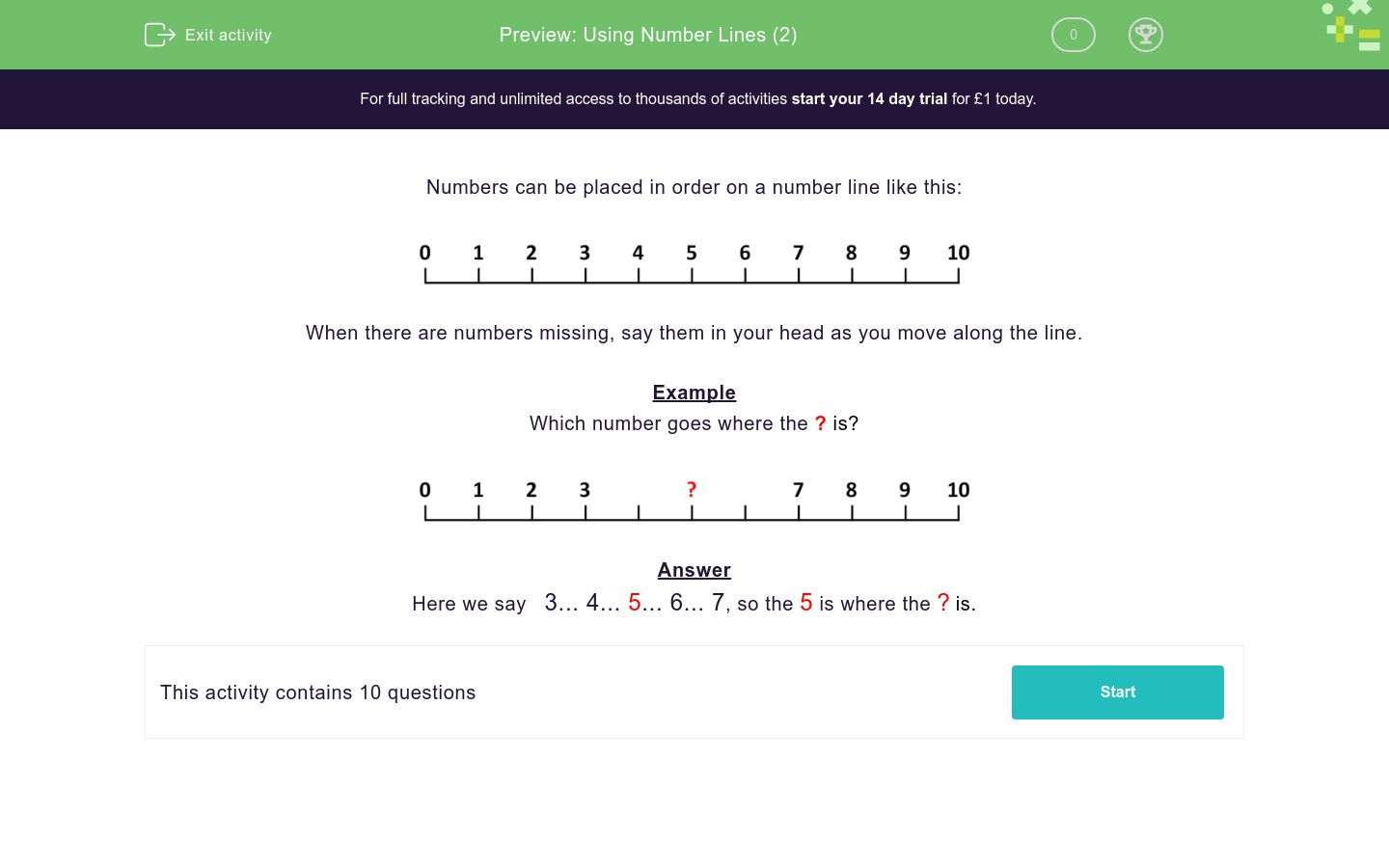# Using Number Lines (2)

In this worksheet, students must identify the missing number on a number line.Key stage:  KS 1

Curriculum topic:   Number: Number and Place Value

Curriculum subtopic:   Represent Numbers to 100

Difficulty level:### QUESTION 1 of 10

Numbers can be placed in order on a number line like this:When there are numbers missing, say them in your head as you move along the line.

Example

Which number goes where the ? is?Here we say   3... 4... 5... 6... 7, so the 5 is where the ? is.

Write the number which goes where the ? is found on this number line.Write the number which goes where the ? is found on this number line.Write the number which goes where the ? is found on this number line.Write the number which goes where the ? is found on this number line.Write the number which goes where the ? is found on this number line.Write the number which goes where the ? is found on this number line.Write the number which goes where the ? is found on this number line.Write the number which goes where the ? is found on this number line.Write the number which goes where the ? is found on this number line.Write the number which goes where the ? is found on this number line.• Question 1

Write the number which goes where the ? is found on this number line.2
EDDIE SAYS
0... 1... 2 ... 3... 4
• Question 2

Write the number which goes where the ? is found on this number line.5
EDDIE SAYS
3... 4... 5 ... 6... 7
• Question 3

Write the number which goes where the ? is found on this number line.6
EDDIE SAYS
4... 5... 6 ... 7... 8
• Question 4

Write the number which goes where the ? is found on this number line.1
EDDIE SAYS
0... 1 ... 2... 3
• Question 5

Write the number which goes where the ? is found on this number line.0
EDDIE SAYS
0... 1... 2... 3... 4
• Question 6

Write the number which goes where the ? is found on this number line.4
EDDIE SAYS
1... 2... 3... 4 ... 5... 6
• Question 7

Write the number which goes where the ? is found on this number line.8
EDDIE SAYS
5... 6... 7... 8 ... 9... 10
• Question 8

Write the number which goes where the ? is found on this number line.7
EDDIE SAYS
4... 5... 6... 7 ... 8... 9
• Question 9

Write the number which goes where the ? is found on this number line.9
EDDIE SAYS
5... 6... 7... 8... 9 ... 10
• Question 10

Write the number which goes where the ? is found on this number line.10
EDDIE SAYS
4... 5... 6... 7... 8... 9... 10
---- OR ----

Sign up for a £1 trial so you can track and measure your child's progress on this activity.

### What is EdPlace?

We're your National Curriculum aligned online education content provider helping each child succeed in English, maths and science from year 1 to GCSE. With an EdPlace account you’ll be able to track and measure progress, helping each child achieve their best. We build confidence and attainment by personalising each child’s learning at a level that suits them.

Get started